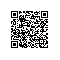# 缓存篇~第七回　Redis实现基于方法签名的数据集缓存（可控更新，分布式数据缓存）

 /// <summary>
/// 表示实现该接口的类型是能够为应用程序提供缓存机制的类型。
/// 这可以有多种实现机制
/// </summary>
public interface ICacheProvider
{
#region Methods
/// <summary>
/// 向缓存中添加一个对象。
/// </summary>
/// <param name="key">缓存的键值，该值通常是使用缓存机制的方法的名称。</param>
/// <param name="valKey">缓存值的键值，该值通常是由使用缓存机制的方法的参数值所产生。</param>
/// <param name="value">需要缓存的对象。</param>
void Add(string key, string valKey, object value);
/// <summary>
/// 向缓存中更新一个对象。
/// </summary>
/// <param name="key">缓存的键值，该值通常是使用缓存机制的方法的名称。</param>
/// <param name="valKey">缓存值的键值，该值通常是由使用缓存机制的方法的参数值所产生。</param>
/// <param name="value">需要缓存的对象。</param>
void Put(string key, string valKey, object value);
/// <summary>
/// 从缓存中读取对象。
/// </summary>
/// <param name="key">缓存的键值，该值通常是使用缓存机制的方法的名称。</param>
/// <param name="valKey">缓存值的键值，该值通常是由使用缓存机制的方法的参数值所产生。</param>
/// <returns>被缓存的对象。</returns>
object Get(string key, string valKey);
/// <summary>
/// 从缓存中移除对象。
/// </summary>
/// <param name="key">缓存的键值，该值通常是使用缓存机制的方法的名称。</param>
void Remove(string key);
/// <summary>
/// 获取一个<see cref="Boolean"/>值，该值表示拥有指定键值的缓存是否存在。
/// </summary>
/// <param name="key">指定的键值。</param>
/// <returns>如果缓存存在，则返回true，否则返回false。</returns>
bool Exists(string key);
/// <summary>
/// 获取一个<see cref="Boolean"/>值，该值表示拥有指定键值和缓存值键的缓存是否存在。
/// </summary>
/// <param name="key">指定的键值。</param>
/// <param name="valKey">缓存值键。</param>
/// <returns>如果缓存存在，则返回true，否则返回false。</returns>
bool Exists(string key, string valKey);
#endregion
}

    /// <summary>
///使用redis方式进行缓存持久化
/// </summary>
internal class RedisCacheProvider : ICacheProvider, IDisposable
{
private readonly IRedisClient _cacheManager = Redis.Client.RedisManager.GetClient();
static byte[] Serialize(object data)
{
BinaryFormatter formatter = new BinaryFormatter();
MemoryStream rems = new MemoryStream();
formatter.Serialize(rems, data);
return rems.GetBuffer();
}
static object Deserialize(byte[] data)
{
BinaryFormatter formatter = new BinaryFormatter();
MemoryStream rems = new MemoryStream(data);
data = null;
return formatter.Deserialize(rems);
}
public void Add(string key, string valKey, object value)
{
byte[] byteValue = Serialize(value);
using (var tbl = _cacheManager.GetTypedClient<Dictionary<string, byte[]>>())
{
Dictionary<string, byte[]> dict = null;
if (tbl.ContainsKey(key))
{
dict = (Dictionary<string, byte[]>)tbl.Lists[key];
dict[valKey] = byteValue;

}
else
{
dict = new Dictionary<string, byte[]>();
}
Remove(key);
}

}

public void Put(string key, string valKey, object value)
{
}

public object Get(string key, string valKey)
{
using (var tbl = _cacheManager.GetTypedClient<Dictionary<string, byte[]>>())
{
if (tbl.ContainsKey(key))
{
Dictionary<string, byte[]> dict = (Dictionary<string, byte[]>)tbl.Lists[key];
if (dict != null && dict.ContainsKey(valKey))
return Deserialize(dict[valKey]);
else
return null;
}
}
return null;

}

public void Remove(string key)
{
using (var tbl = _cacheManager.GetTypedClient<Dictionary<string, byte[]>>())
{
tbl.Lists[key].RemoveAll();
}
}

public bool Exists(string key)
{
using (var tbl = _cacheManager.GetTypedClient<Dictionary<string, byte[]>>())
{
return tbl.ContainsKey(key);
}
}

public bool Exists(string key, string valKey)
{
using (var tbl = _cacheManager.GetTypedClient<Dictionary<string, byte[]>>())
{
return tbl.ContainsKey(key) &&
((System.Collections.Generic.Dictionary<string, byte[]>)tbl.Lists[key]).ContainsKey(valKey);
}
}

public void Dispose()
{
_cacheManager.Dispose();
}
}使用钉钉扫一扫加入圈子
+ 订阅